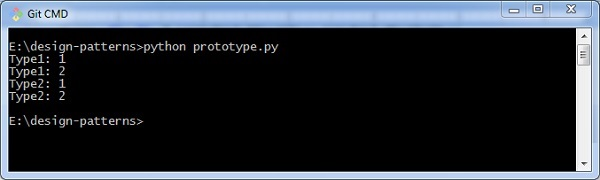# Python Design Patterns - Prototype

Prototype design pattern helps to hide the complexity of the instances created by the class. The concept of the existing object will differ with that of the new object, which is created from scratch.

The newly copied object may have some changes in the properties if required. This approach saves time and resources that go in for the development of a product.

## How to implement a prototype pattern?

Let us now see how to implement a prototype pattern.

```import copy

class Prototype:

_type = None
_value = None

def clone(self):
pass

def getType(self):
return self._type

def getValue(self):
return self._value

class Type1(Prototype):

def __init__(self, number):
self._type = "Type1"
self._value = number

def clone(self):
return copy.copy(self)

class Type2(Prototype):

""" Concrete prototype. """

def __init__(self, number):
self._type = "Type2"
self._value = number

def clone(self):
return copy.copy(self)

class ObjectFactory:

""" Manages prototypes.
Static factory, that encapsulates prototype
initialization and then allows instatiation
of the classes from these prototypes.
"""

__type1Value1 = None
__type1Value2 = None
__type2Value1 = None
__type2Value2 = None

@staticmethod
def initialize():
ObjectFactory.__type1Value1 = Type1(1)
ObjectFactory.__type1Value2 = Type1(2)
ObjectFactory.__type2Value1 = Type2(1)
ObjectFactory.__type2Value2 = Type2(2)

@staticmethod
def getType1Value1():
return ObjectFactory.__type1Value1.clone()

@staticmethod
def getType1Value2():
return ObjectFactory.__type1Value2.clone()

@staticmethod
def getType2Value1():
return ObjectFactory.__type2Value1.clone()

@staticmethod
def getType2Value2():
return ObjectFactory.__type2Value2.clone()

def main():
ObjectFactory.initialize()

instance = ObjectFactory.getType1Value1()
print "%s: %s" % (instance.getType(), instance.getValue())

instance = ObjectFactory.getType1Value2()
print "%s: %s" % (instance.getType(), instance.getValue())

instance = ObjectFactory.getType2Value1()
print "%s: %s" % (instance.getType(), instance.getValue())

instance = ObjectFactory.getType2Value2()
print "%s: %s" % (instance.getType(), instance.getValue())

if __name__ == "__main__":
main()
```

### Output

The above program will generate the following output −The output helps in creating new objects with the existing ones and it is clearly visible in the output mentioned above.